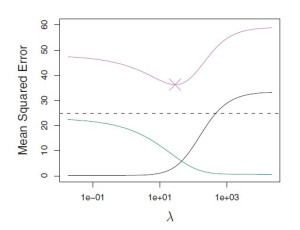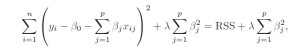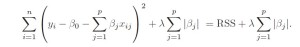# Shrinkage Methods in Linear Regression

Ever have a question that, “Why is Linear Regression giving me such good accuracy on the training set but a low accuracy on the test set in spite of adding all the available dependent features to the model?”

The question above seems inexplicable to many people but is answered by a concept called overfitting in which your model, in addition to learning the data, also learns the noise present in it. Hence learning the training points, a bit too perfectly.

How do you solve it?

This is where shrinkage methods (also known as regularization) come in play. These methods apply a penalty term to the Loss function used in the model. Minimizing the loss function is equal to maximizing the accuracy. To understand this better, we need to go into the depths of Loss function in Linear Regression.

Linear Regression uses Least Squares to calculate the minimum error between the actual values and the predicted values. The aim is to minimize the squared difference between the actual and predicted values to draw the best possible regression curve for the best prediction accuracy.

Now, what does shrinking do?

Shrinking the coefficient estimates significantly reduces their variance. When we perform shrinking, we essentially bring the coefficient estimates closer to 0.

The need for shrinkage method arises due to the issues of underfitting or overfitting the data. When we want to minimize the mean error (Mean Squared Error(MSE) in case of Linear Regression), we need to optimize the bias-variance trade-off.

The bias-variance trade-off indicates the level of underfitting or overfitting of the data with respect to the Linear Regression model applied to it. A high bias-low variance means the model is underfitted and a low bias-high variance means that the model is overfitted. We need to trade-off between bias and variance to achieve the perfect combination for the minimum Mean Squared Error as shown by the graph below.In this figure, the green curve is variance, the black curve is squared bias and the purple curve is the MSE. Lambda is the regularization parameter which will be covered later.

How do we use shrinking methods?

The best known shrinking methods are Ridge Regression and Lasso Regression which are often used in place of Linear Regression.
Ridge Regression, like Linear Regression, aims to minimize the Residual Sum of Squares(RSS) but with a slight change. As we know, Linear Regression estimates the coefficients using the values that minimize the following equation:Ridge Regression adds a penalty term to this, lambda, to shrink the coefficients to 0 :Ridge Regression’s advantage over Linear Regression is that it capitalizes on the bias-variance trade-off. As λ increases, the coefficients shrink more towards 0.

Ridge Regression has a major disadvantage that it includes all p predictors in the output model regardless of the value of their coefficients which can be challenging for a model with huge number of features. This disadvantage is overcome by Lasso Regression which performs variable selection. Lasso Regression uses L-1 penalty as compared to Ridge Regression’s L-2 penalty which instead of squaring the coefficient, takes its absolute value as shown below :Ridge Regression brings the value of coefficients close to 0 whereas Lasso Regression forces some of the coefficient values to be exactly equal to 0. It is important to optimize the value of λ in Lasso Regression as well to reduce the MSE error.

Take Away

In conclusion, shrinkage methods provide us with better Regression Models as they minimize the possibility of overfitting or underfitting the data by adding a penalty term to the RSS. Hence it safely removes the misconception that if a Linear Regression model predicts with a good accuracy on a training set then it will also predict with the same accuracy on the test set.
We now know that there are better methods than simple Linear Regression in the form of Ridge Regression and Lasso Regression which account for the underfitting and overfitting of data.

Interested in knowing more on such niche techniques? Check out http://research.busigence.com